# # 【深度学习】：《100天一起学习PyTorch》模型评估和选择（上）：欠拟合和过拟合

+关注继续查看
• ?本文收录于【深度学习】：《PyTorch入门到项目实战》专栏，此专栏主要记录如何使用PyTorch实现深度学习笔记，尽量坚持每周持续更新，欢迎大家订阅！
• ?个人主页：JoJo的数据分析历险记
• ?个人介绍：小编大四统计在读，目前保研到统计学top3高校继续攻读统计研究生
• ?如果文章对你有帮助，欢迎?关注、?点赞、?收藏、?订阅专栏@TOC

# 1.基本概念

## 1.1训练误差和泛化误差

• 训练误差：模型在训练集上的误差
• 泛化误差：模型误差的期望

## 1.2训练集、验证集和测试集

• 训练集：用于训练模型，得到模型参数
• 验证集：用于选择模型，调整超参数
• 测试集：用于评估模型
 用一个很形象的比喻就是:训练集相当于平时的练习，验证集相当于平时的小测验，测试集相当于期末考试。首先要保证平时练习的练习正确率较高，才能在期末考试中拿到较好的成绩。但是如果作弊看了练习题的答案，那么这个时候平时的练习会有较高的正确率，但是期末考试没有答案抄就拿不到好成绩了，这时就需要平时的小测验来验证一下你的学习成果，来避免你是因为偷看了练习答案从而有有了较高的正确率。 在训练数据时，我不希望用到测试集的数据，因为这样的话测试集得到的评估结果是很容易过拟合的。因此我们需要将数据集分为训练集、验证集和测试集，但是在实际应用是，验证集和测试集往往区分的不是很清楚。因此，很多时候，在实际中只设置了训练集和验证集。因此，我们在后续主要关注验证集的误差。 ## 1.3 交叉验证 我们讨论了训练误差和验证误差。我们常常用交叉验证的方法来计算验证误差： - **留一法交叉验证** 留一法交叉验证，每次将一个样本作为验证集，剩下的n-1个样本作为训练集:${(x_2,y_2),...,(x_n,y_n)}$。拟合模型。如下图所示： ![在这里插入图片描述](https://ucc.alicdn.com/images/user-upload-01/9fe11051c43c4440868d26392202c75c.png#pic_center) 我们相当于做了n次模型训练，然后将这n次拟合的平均验证误差来估计某一个具体模型的验证误差。第一次训练得到的验证误差为：$MSE_1=(y_1-\hat{y}_1)^2$。重复n次得到：$MSE_2,...,MSE_n$。最后我们取平均值得到LOOCV估计的测试MSE: $$CV_{(n)}=\frac{1}{n}\sum_{i=1}^{n}MSE_i.$$ - **K折交叉验证** K折交叉验证的思路是将数据集随机平均的分为K组。第一组作为验证集，剩下的k-1组作为训练集。当k=n时，留一法交叉验证可以看做是K折交叉验证。和留一法交叉验证类似，$MSE_1$可以看做是第一次训练时，验证组的平均误差。重复k次，我们可以得到k-折交叉验证的验证误差： $$CV_{(k)}=\frac{1}{k}\sum_{i=1}^{k}MSE_i.$$ 下图给出了5折交叉验证的示意图： ![在这里插入图片描述](https://ucc.alicdn.com/images/user-upload-01/6d818abc32cb4553934ee8c6d7f1796f.png#pic_center) ## 1.4模型复杂度 在得到训练的模型后，计算其训练误差，验证误差。往往会出现两种情况，一种是过拟合，一种是欠拟合 - 欠拟合(underfitting)：欠拟合是指模型在训练集上表现的也不好，模型不能很好的拟合训练集 - 过拟合(overfitting)：模型在训练集上表现很好，但是在测试集上表现的较差 - 正则化(regularization)：正则化可以用于处理过拟合问题 当模型出现欠拟合时候，我们可以考虑使用更复杂的模型来进行训练，当模型过拟合时，需要减少模型的复杂度。具体关系如下图所示： ![](https://ucc.alicdn.com/images/user-upload-01/img_convert/f27333e005563f2b995487e0ee9b05bb.png#pic_center) 一般来说，当数据集很多时，使用较复杂的模型；当数据集较少时，使用交简单的模型。 下面我们以多项式回归为例来具体看看这些指标情况 # 2. 多项式回归 经过上述一些概念的介绍，下面通过一个多项式的具体例子来看一下,首先多项式回归定义如下： $$y = \beta_0 + \beta_1X+\beta_2X^2+\beta_3X^3+...+\beta_nX^n$$ ==当$\beta_2,...,\beta_n$都为0时，就是一个简单的一元线性回归，因此高次多项式是可以包含低次多项式回归的。==说明高次多项式模型更复杂。下面我们以一个三次多项式的数据为例，分别拟合不同的多项式回归模型，观察其训练误差和验证误差的情况 python import math import numpy as np import torch from torch import nn from torch.utils import data from IPython import display  生成一个模拟数据集，其真实的关系是三次多项式回归 python max_degree = 20 # 多项式的最大阶数 n_train, n_test = 100, 100 # 训练和测试数据集大小 true_w = np.zeros(max_degree) # 设置w true_w[0:4] = np.array([5.1, 1.2, -3.1, 5.1]) features = np.random.normal(size=(n_train + n_test, 1)) np.random.shuffle(features) poly_features = np.power(features, np.arange(max_degree).reshape(1, -1)) for i in range(max_degree): poly_features[:, i] /= math.gamma(i + 1) # labels的维度:(n_train+n_test,) labels = np.dot(poly_features, true_w) labels += np.random.normal(scale=0.1, size=labels.shape)  下面将多维数组转换为张量（tensor） python true_w, features, poly_features, labels = [torch.tensor(x, dtype= torch.float32) for x in [true_w, features, poly_features, labels]]  接下来需要先定义一些基本的函数，大家可以直接下载d2l库导入，在沐神的教材上都有，但是有的时候安装d2l报错，因此如果大家不想安装d2l的话，可以参考一下下面这些函数，大家也可以自己将这些函数写入自己的包中方便导入。 python # 定义数据迭代器函数 def load_array(data_arrays, batch_size, is_train=True): """构造一个PyTorch数据迭代器""" dataset = data.TensorDataset(*data_arrays)#将数据转换为tensor return data.DataLoader(dataset, batch_size, shuffle=is_train)  python # 定义一个类来接收变量 class Accumulator: #@save """在n个变量上累计""" def __init__(self, n): self.data = [0.0] * n def add(self, *args): self.data = [a + float(b) for a, b in zip(self.data, args)] def reset(self): self.data = [0.0] * len(self.data) def __getitem__(self, idx): return self.data[idx]  python # 定义准确率函数 def accuracy(y_hat, y): #@save """计算预测正确的数量""" if len(y_hat.shape) > 1 and y_hat.shape > 1: y_hat = y_hat.argmax(axis=1) cmp = y_hat.type(y.dtype) == y return float(cmp.type(y.dtype).sum())  python # 计算误差函数 def evaluate_loss(net, data_iter, loss): #@save """评估给定数据集上模型的损失""" metric = Accumulator(2) # 损失的总和,样本数量 for X, y in data_iter: out = net(X) y = y.reshape(out.shape) l = loss(out, y) metric.add(l.sum(), l.numel()) return metric / metric  python # 训练函数 def train_epoch(net, train_iter,loss,updater): """三个变量，训练损失，训练准确度，样本数""" net.train() metric = Accumulator(3) for X,y in train_iter: y_hat = net(X) l = loss(y_hat,y) if isinstance(updater, torch.optim.Optimizer):#如果是pytorch内置优化器 updater.zero_grad() l.mean().backward() updater.step() else: """自己定义的优化器""" l.sum().backward() updater(X.shape) metric.add(float(l.sum()),accuracy(y_hat,y),y.numel()) return metric/metric, metric/metric  python # 定义坐标轴函数 def set_axes(axes, xlabel, ylabel, xlim, ylim, xscale, yscale, legend): """设置matplotlib的轴""" axes.set_xlabel(xlabel) axes.set_ylabel(ylabel) axes.set_xscale(xscale) axes.set_yscale(yscale) axes.set_xlim(xlim) axes.set_ylim(ylim) if legend: axes.legend(legend) axes.grid() # 定义保存函数 def use_svg_display(): #@save """使用svg格式在Jupyter中显示绘图""" display.set_matplotlib_formats('svg')  python # 定义一个动画绘制类 class Animator: #@save """在动画中绘制数据""" def __init__(self, xlabel=None, ylabel=None, legend=None, xlim=None, ylim=None, xscale='linear', yscale='linear', fmts=('-', 'm--', 'g-.', 'r:'), nrows=1, ncols=1, figsize=(3.5, 2.5)): # 增量地绘制多条线 if legend is None: legend = [] plt.show() self.fig, self.axes = plt.subplots(nrows, ncols, figsize=figsize) if nrows * ncols == 1: self.axes = [self.axes, ] # 使用lambda函数捕获参数 self.config_axes = lambda: set_axes( self.axes, xlabel, ylabel, xlim, ylim, xscale, yscale, legend) self.X, self.Y, self.fmts = None, None, fmts def add(self, x, y): # 向图表中添加多个数据点 if not hasattr(y, "__len__"): y = [y] n = len(y) if not hasattr(x, "__len__"): x = [x] * n if not self.X: self.X = [[] for _ in range(n)] if not self.Y: self.Y = [[] for _ in range(n)] for i, (a, b) in enumerate(zip(x, y)): if a is not None and b is not None: self.X[i].append(a) self.Y[i].append(b) self.axes.cla() for x, y, fmt in zip(self.X, self.Y, self.fmts): self.axes.plot(x, y, fmt) self.config_axes() display.display(self.fig) display.clear_output(wait=True)  python # 定义训练函数 def train(train_features, test_features, train_labels, test_labels, num_epochs=400): loss = nn.MSELoss(reduction='none')#设置损失函数为MSE input_shape = train_features.shape[-1] # 不设置偏差，因为我们已经在多项式中已经设置好了 net = nn.Sequential(nn.Linear(input_shape, 1, bias=False))#定义线性神经网络 batch_size = min(10, train_labels.shape)#确定batch train_iter = load_array((train_features, train_labels.reshape(-1,1)), batch_size)#训练集 test_iter = load_array((test_features, test_labels.reshape(-1,1)) ,batch_size)#测试集 trainer = torch.optim.SGD(net.parameters(), lr=0.01)#训练集，使用SGD训练模型 animator = Animator(xlabel='epoch', ylabel='loss', yscale='log', xlim=[1, num_epochs], ylim=[1e-3, 1e2], legend=['train', 'test'])#图形相关设置 for epoch in range(num_epochs): train_epoch(net, train_iter, loss, trainer) if epoch == 0 or (epoch + 1) % 20 == 0: animator.add(epoch + 1, (evaluate_loss(net, train_iter, loss),#计算训练误差并绘制 evaluate_loss(net, test_iter, loss)))#计算测试误差并绘制 print('weight:', net.weight.data.numpy())  ## 2.1 三次多项式回归（正常拟合） 因为我们生成的数据集是3次多项式回归得到的，因此使用三次多项式回归拟合结果会很精确 python train(poly_features[:n_train, :4], poly_features[n_train:, :4], labels[:n_train], labels[n_train:])  weight: [[ 5.1068187 1.2157811 -3.1099443 5.064199 ]] ![png](https://ucc.alicdn.com/images/user-upload-01/img_convert/706233afe1b4fd80fbcbbee3ae9d03d1.png#pic_center) 可以看出，随着训练次数的增加，训练误差和验证误差都不断下降到小于0.01，并且验证误差和验证误差基本一致 ## 2.2 一元线性回归（underfitting） 下面我们使用一元线性回归来拟合数据，由于我们知道真实的数据集是三次关系的，此时使用一元线性回归无法进行精确拟合，会导致模型的bias较大，训练误差和验证误差都很大 python # 从多项式特征中选择前2个维度，即1和x train(poly_features[:n_train, :2], poly_features[n_train:, :2], labels[:n_train], labels[n_train:])  weight: [[3.8188436 3.0646155]] ![png](https://ucc.alicdn.com/images/user-upload-01/img_convert/3dded050ea8a23bc3ea55a5bd7d63ddd.png#pic_center) 从上图可以看出，和我们预计得到的结果一致，由于模型太简单，连训练集上也不能很好的拟合，导致训练误差和验证误差都很大 ## 2.3 10次多项式（过拟合） 下面我们使用10次多项式来进行拟合，由于模型的复杂度太高，会导致模型出现过拟合，验证集上的误差会随着训练次数增加会先下降再上升 python # 从多项式特征中选取所有维度 train(poly_features[:n_train, :11], poly_features[n_train:, :11], labels[:n_train], labels[n_train:], num_epochs=500)  weight: [[ 5.0872297 1.2546227 -2.9732502 4.719495 -0.47507587 1.4278368 -0.05434499 0.30877623 0.28959352 0.18821514 0.06768304]] ![png](https://ucc.alicdn.com/images/user-upload-01/img_convert/dcf3a21d5ba506985a640472c8783f8b.png#pic_center) 从上图可以看出，和我们预计的一致，验证误差先减小后增大，如果我们提前结束训练的话，能够得到还不错的结果，这种后续中会介绍 # 3.总结10 0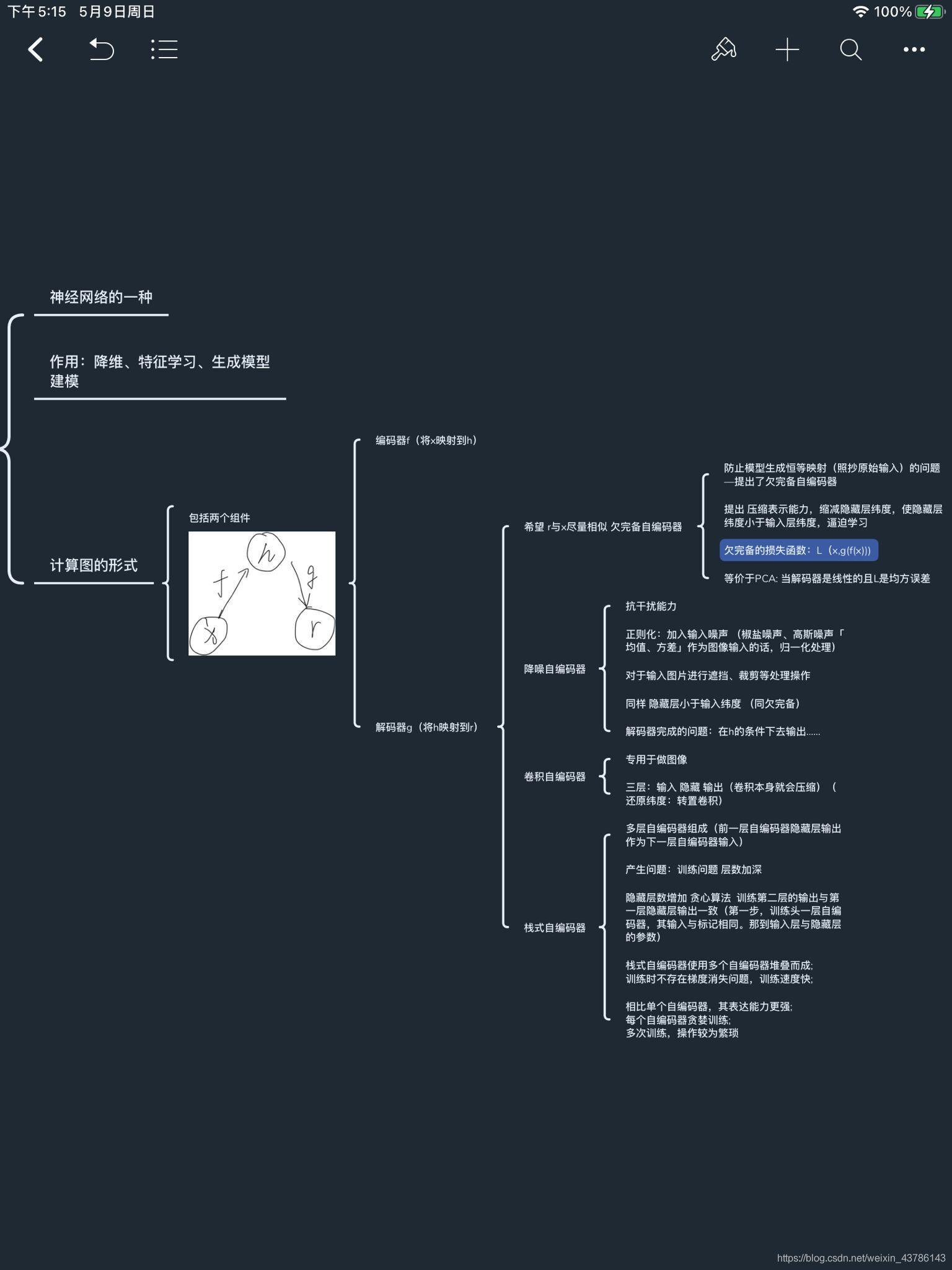43 0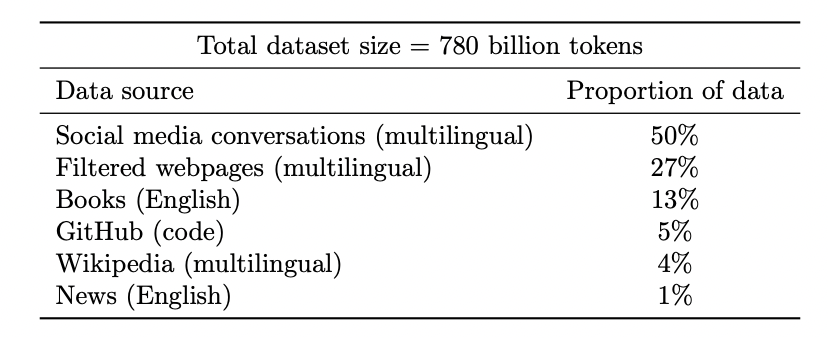18 0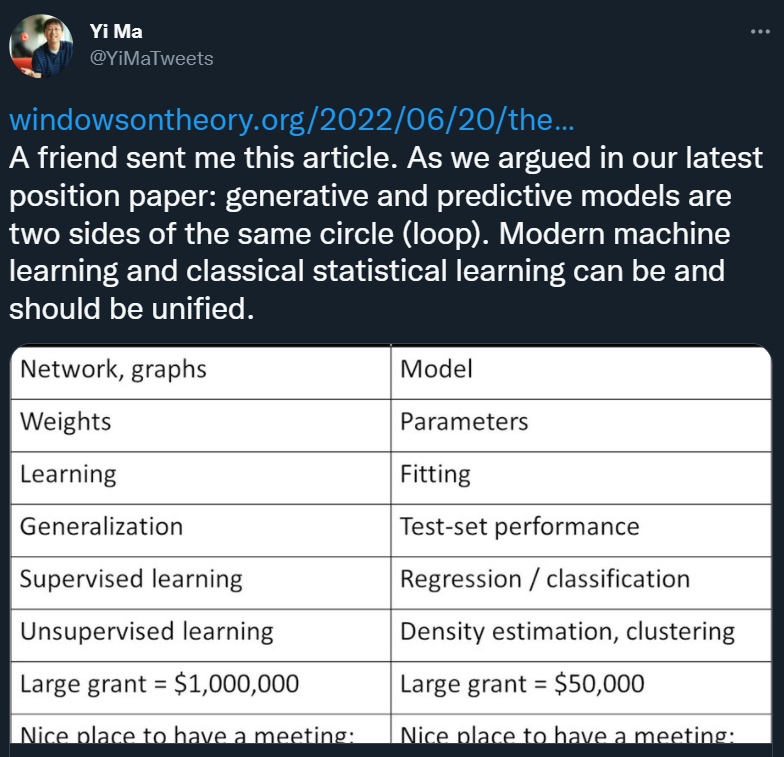16 0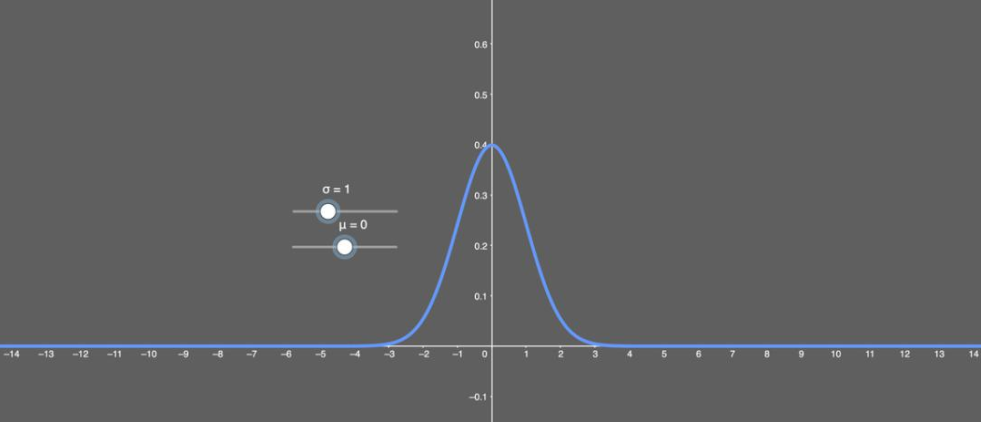【深度学习】2、Pytorch自行实现常见的11个激活函数的Fashion Minist项目实践对比（你需要的这里都有了！）（三）
【深度学习】2、Pytorch自行实现常见的11个激活函数的Fashion Minist项目实践对比（你需要的这里都有了！）（三）
30 0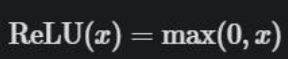【深度学习】2、Pytorch自行实现常见的11个激活函数的Fashion Minist项目实践对比（你需要的这里都有了！）（二）
【深度学习】2、Pytorch自行实现常见的11个激活函数的Fashion Minist项目实践对比（你需要的这里都有了！）（二）
23 0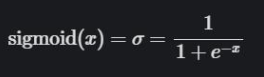【深度学习】2、Pytorch自行实现常见的11个激活函数的Fashion Minist项目实践对比（你需要的这里都有了！）（一）
【深度学习】2、Pytorch自行实现常见的11个激活函数的Fashion Minist项目实践对比（你需要的这里都有了！）（一）
18 0
ModelScope旨在打造下一代开源的模型即服务共享平台，为泛AI开发者提供灵活、易用、低成本的一站式模型服务产品，让模型应用更简单！

【算法实战】9. 线性回归算法335